## Logic Gates-

Before you go through this article, make sure that you have gone through the previous article on Logic Gates.

We have discussed-

• Logic gates are the basic building blocks of any digital circuit.
• There are 3 basic logic gates- AND, NOT, OR.
• Logic gates are classified as-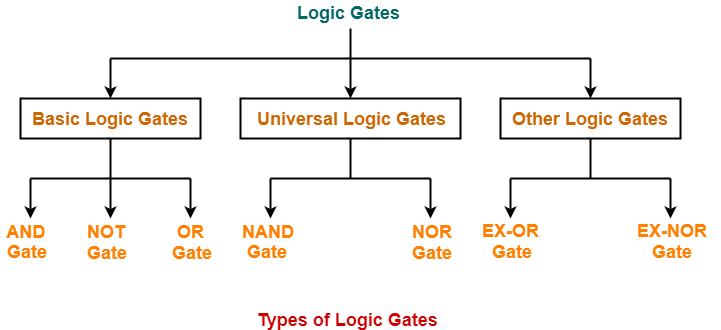## Universal Logic Gates-

 Universal logic gates are the logic gates that are capable of implementing any Boolean function without requiring any other type of gate.

They are called as “Universal Gates” because-

• They can realize all the binary operations.
• All the basic logic gates can be derived from them.

They have the following properties-

• Universal gates are not associative in nature.
• Universal gates are commutative in nature.

There are following two universal logic gates-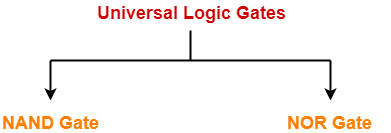1. NAND Gate
2. NOR Gate

## 1. NAND Gate-

• A NAND Gate is constructed by connecting a NOT Gate at the output terminal of the AND Gate.
• The output of NAND gate is high (‘1’) if at least one of its inputs is low (‘0’).
• The output of NAND gate is low (‘0’) if all of its inputs are high (‘1’).

### Logic Symbol-

The logic symbol for NAND Gate is as shown below-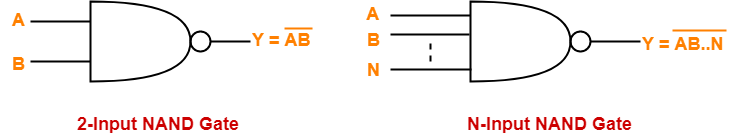### Truth Table-

The truth table for NAND Gate is as shown below-

 A B Y = (A.B)’ 0 0 1 0 1 1 1 0 1 1 1 0

### Timing Diagram-

The timing diagram for NAND Gate is as shown below-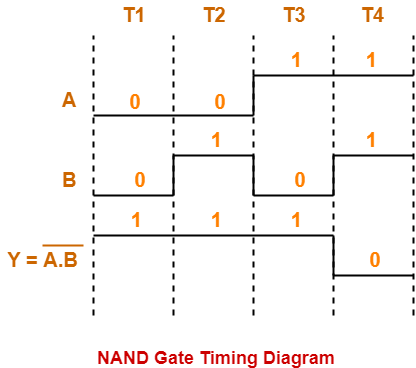## 2. NOR Gate-

• A NOR Gate is constructed by connecting a NOT Gate at the output terminal of the OR Gate.
• The output of OR gate is high (‘1’) if all of its inputs are low (‘0’).
• The output of OR gate is low (‘0’) if any of its inputs is high (‘1’).

### Logic Symbol-

The logic symbol for NOR Gate is as shown below-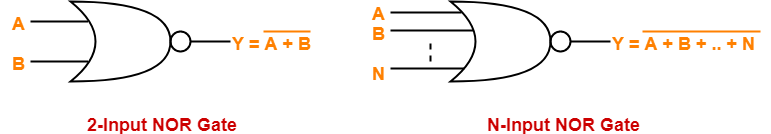### Truth Table-

The truth table for NOR Gate is as shown below-

 A B Y = A + B 0 0 1 0 1 0 1 0 0 1 1 0

### Timing Diagram-

The timing diagram for NOR Gate is as shown below-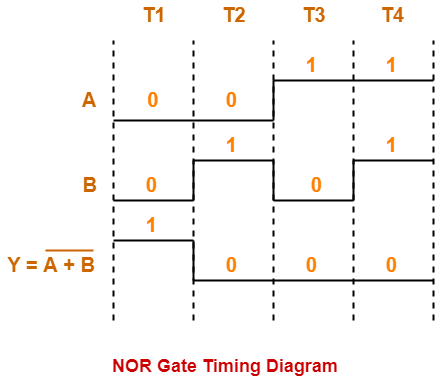To gain better understanding about Universal Logic Gates,

Watch this Video Lecture

Next Article- Alternative Logic Gates

Get more notes and other study material of Digital Design.

Watch video lectures by visiting our YouTube channel LearnVidFun.